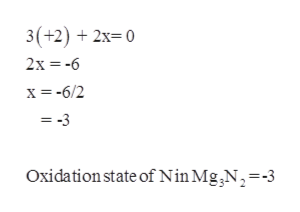# Give the oxidation numbers for the specified atoms in the following molecules and ions.(a) Mg3N2   N:(b) CaC2    C:(c) ZnO22−   O: (d) NaBH4   B:(e) C2O42−   C:(f) WO42−    W:

Question
48 views

Give the oxidation numbers for the specified atoms in the following molecules and ions.
(a) Mg3N2   N:

(b) CaC2    C:

(c) ZnO22−   O:

(d) NaBH4   B:

(e) C2O42−   C:

(f) WO42−    W:

check_circle

Step 1

(a)Mg3N2:

N: Assume that the oxidation state of N = x

Oxidation sate of Mg = +2help_outlineImage Transcriptionclose3(+2)2x- 0 2x -6 x-6/2 =-3 Oxidation state of Nin Mg ,N, = -3 fullscreen
Step 2

(b)CaC2:

C: Assume that the oxidation state of C = x

Oxidation sate of Ca = +2

Step 3

(c)ZnO22-:

O: Assume that the oxidation state of O = x

...

### Want to see the full answer?

See Solution

#### Want to see this answer and more?

Solutions are written by subject experts who are available 24/7. Questions are typically answered within 1 hour.*

See Solution
*Response times may vary by subject and question.
Tagged in
ScienceChemistry

### Physical Chemistry